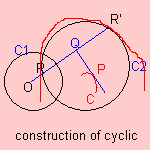# cyclic

## mainwhere r = f(φ) is the polar equation of the pedal of the curve to be derived.

Given a curve C and a fixed point O.
Let O be the pedal point, then select a point P of curve C and draw its pedal Q.
Then construct points R and R' on OQ for which OR*OR' = a, and OR+OR'=2OQ. The collection of the points R and R' form the cyclic of C.Graphically you can see that the construction can be seen as in inversion: define the inversion circle C1 as the circle with center O and radius √a. Then construct a circle C2 with center P, which is orthogonal to the inversion circle C1.
Then the envelope of the circles C2 is the cyclic of curve C.
The parameter a is called the power (of inversion).

Parameter 'a' being unequal zero, the cyclic is an anallagmatic curve.
For parameter 'a' being zero, the cyclic is said to be equal to an orthotomic. Then it is called rational.

Some cyclic relations are the following:
 curve C1 center of inversion cyclic of C1 conic center bicircular quartic hyperbola center ovale of Cassini parabola focus circular cubic

Generalizing the notion to a surface you get the cyclid.